The Sundial Primer created by Carl SabanskiThe Sundial Primer Index
 Analemmic Sundial Analemmic Sundial: this term has sometimes been used to describe dials which have an analemma-shape gnomon, or analemmas on the hour lines, enabling them to read mean time.Analemma: in modern usage it is a graphical plot of the Equation of Time on one axis and the sun's declination on the other. In appearance, a tall thin figure eight. The dates of various points around the curve are often shown. The shadow of a point falling onto an arbitrary plane at the same clock time each day will trace out an analemma over the course of a year.  Normally seen on the noon line of a dial, but can be on any hour line. The exact appearance of the analemma will depend on the plane upon which it is projected. The gnomon of the equatorial ring sundial can designed to compensate for the Equation of Time. This is done by creating an analemmic style that has a built in correction for the Equation of Time. This is possible to do because the hour lines for this dial are equally spaced at 15°.  To create this style an analemma must be determined proportional to the size of the equatorial ring being designed. Knowing the radius "r" of the equatorial ring, co-ordinates for the analemma can be calculated as follows.x = r tan EoT°y = r tan DEC where DEC is the sun's declination at noon and EoT is the Equation of Time at noon expressed in degrees as follows:EoT° = EoTs / 240where EoTs is the Equation of Time in seconds and 1° is equal to 4 minutes (240 seconds).The sun's declination and Equation of Time vary slightly from year to year at any given time. The values used can be an average over a couple of years between leap years or the mean year. Figure 1 illustrates an analemma that can be used to create an analemmic style.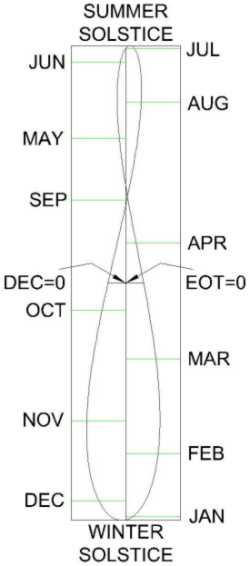Figure 1: Analemma for Equatorial Sundial (SONNE/CAD) The data for this analemma was obtained from the sundial design program SONNE. This program outputs a text file of the sun's declination and Equation of Time at noon for any selected year. The text files were then imported into a spreadsheet where they were used to calculate the x and y co-ordinates for the analemma. The co-ordinates were saved as a comma delimited file and were used with a line command in a CAD program to draw a series of line segments that became the image shown in Figure 1. Click here if you would like to download this analemma in PDF format.Click here if you would like to download a spreadsheet that calculates the x and y co-ordinates for the analemma for any radius r. The calculations are based on average values of the Equation of Time and the sun's declination for the period 2000 to 2047.The following describes two analemmic styles that can be created from the analemma shown in Figure 1. In both cases the origin of the analemma, determined by the point where the declination and EoT are both zero, must be located at the centre of the equatorial ring.  The y axis also lies along the polar axis of the equatorial sundial. The time is indicated along the appropriate edge as shown above. The first style is an analemmic body or two as illustrated in Figure 2. Because the analemma is not symmetrical about the polar (y) axis where the Equation of Time equals zero it is not possible to create a 3-dimensional shape from this image. There are two things that can be done. The analemma can be adjusted to be symmetrical about the y axis so that it can be revolved about the polar axis to create a solid body.  This will introduce an error throughout the year. The second option is to break the analemma at the summer and winter solstices and create two curves. These curves, when revolved about the polar axis will create two analemmic bodies as shown in Figure 2. One is used during the period from December to June and the other is used during the other half of the year.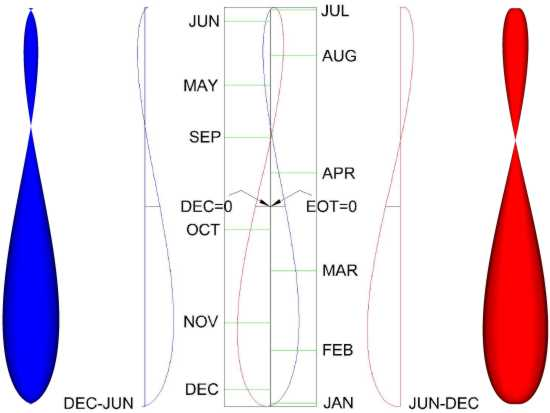Figure 2: Analemmic Shaped BodiesAll these bodies do pose some problems. In all cases there is the requirement to mount these bodies in position whether on a wire or a rod. Portions of the analemmic bodies, certainly in the regions where the Equation of Time approaches zero, will be lost. If two bodies are created they must be changed twice a year and it may be difficult to position them accurately. If an adjusted analemmic body is used, even though it will always have an error, it needs to be positioned only once.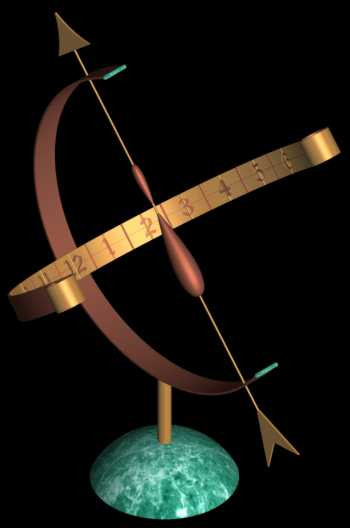For an image complete with shadow click here. There is a second type of analemmic style that does not have some of the inherent problems of the style described above. The complete analemma can be cut into a flat plate and will include all points for the entire year. This analemmic style is illustrated in Figure 3. The plate is positioned on the polar axis as described above. To read the time the plate is rotate until it directly faces the sun. This is determined when the analemma shape is the smallest. The time is read where the appropriate edge intersects a line located along the centre of the equatorial ring.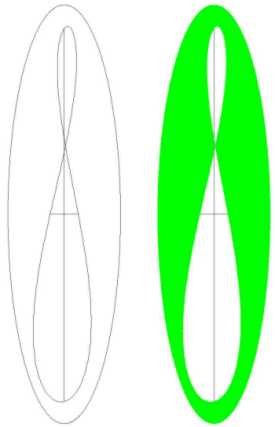Figure 3: Analemmic Shaped Plate For both the dials described above the corrections remaining are for longitude and Daylight Saving Time. These corrections can be made if the dial is designed with an equatorial ring that can be rotated about the polar axis or by shifting the hour lines on the equatorial ring.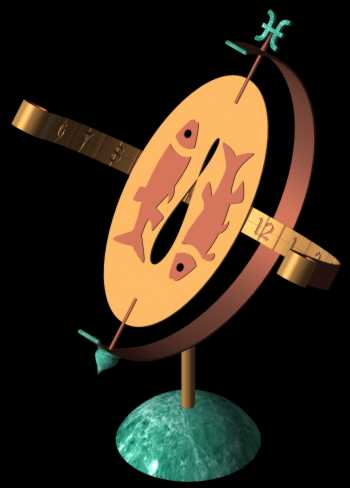For an image complete with shadow click here.